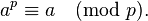Problem Statement

We are given cells. Initially all cells are completely white. We are provided with two colors white and black. We are also given two arrays L[ ] and R[ ]. At each iteration i, paint all the cells from L[i-1] to R[i-1] with either black or white. We have to find total number of configurations possible. Two configurations are different if at least on cell in white in one configuration and black in another.

You can find a detailed problem description here.

Hackerrank Nov Challenge – Power of Large Numbers

PROBLEM STATEMENT

In a nutshell, we have a^b mod 10^9 + 7 ( ^ denotes power )

Range of a & b: 1 <= a,b <= 10^100000

PRE – REQUISITES

FERMAT’S THEOREM:

The theorem stats that, if is a prime number, then for any arbitrary integer